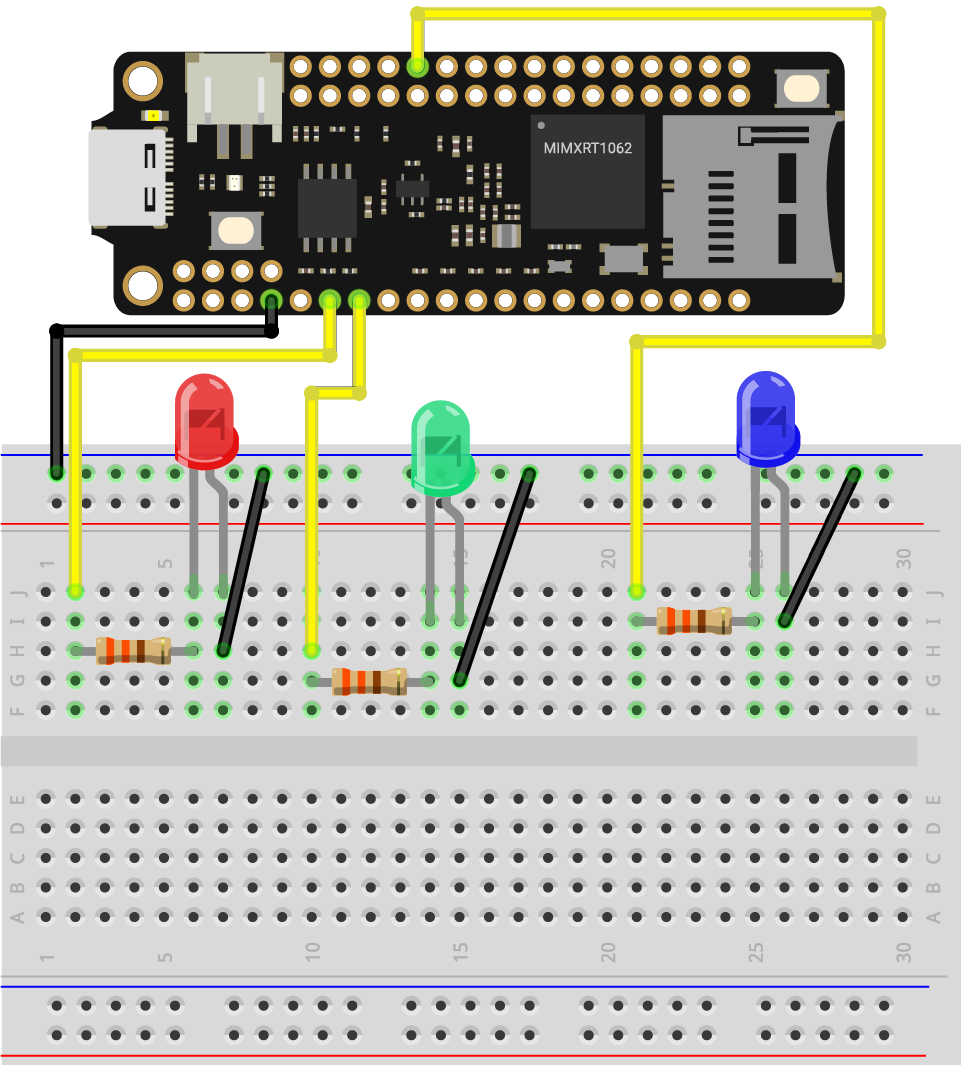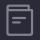# LEDs brightness control

This demo is similar to the previous PWM brightness control, but you will make 3 LEDs to do so one by one.

## What you need​

• SwiftIO Feather (or SwiftIO board)
• 3x LEDs
• 3x 330ohm resistors
• Jumper wires

## Circuit​

1. Plug the three LEDs onto the breadboard.
2. Connect the short leg of the red LED to pin GND and the long leg to PWM0A through a resistor.
3. Connect the short leg of the green LED to pin GND and the long leg PWM1A through a resistor.
4. Connect the short leg of the blue LED to pin GND and the long leg PWM2B through a resistor.## Example code​

You can find the example code at the bottom left corner of IDE:/ `SimpleIO` / `LEDsBrightnessControl`.

``// Change the LED state every second by setting the interrupt.// Import the library to enable the relevant classes and functions.import SwiftIO// Import the board library to use the Id of the specific board.import MadBoard// Initialize the pins the LEDs are connected to and put them in a array.let red = PWMOut(Id.PWM0A)let green = PWMOut(Id.PWM1A)let blue = PWMOut(Id.PWM2B)let leds = [red, green, blue]// Declare a variable to store the value of duty cycle.var value: Float = 0.0// Change the brightness of each LED over and over again.while true {    // Iterate each LED in the array.     // This allows the LED to go through the following process one by one.    for led in leds {        // Brighten the LED in two seconds.        while value <= 1.0 {            led.setDutycycle(value)            sleep(ms: 20)            value += 0.01        }        // Keep the value of duty cycle between 0.0 and 1.0.        value = 1.0        // Dimming the LED in two seconds.        while value >= 0 {            print(value)            led.setDutycycle(value)            sleep(ms: 20)            value -= 0.01        }        // Keep the value of duty cycle between 0.0 and 1.0.        value = 0.0    }}``

## Code analysis​

``let leds = [red, green, blue]``

There are 3 LEDs in total. They all repeat the same operations. So you can put them in an array.

An array is a collection of ordered values of the same type. To access its item, you can use its index. The index starts from 0. So if you want to access red led, it's `leds`.

``for led in leds {}``

Then you can use `for-in` loop to iterate over the array. That's why you create it. You can avoid repeating the same code many times and thus simplify the code.

`led` represents each item in the array `leds`, and you can name it as you like. In the loop, the first item will execute the code in the brackets. After that, the second one repeats the task. The task will repeat several times until all items finish it.

## Reference​

PWMOut - set the PWM signal.